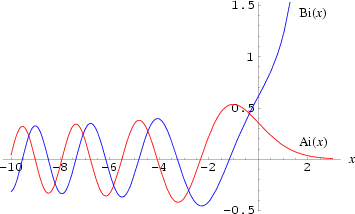•http://facebook.com/
•https://www.google.com/accounts/o8/id
•https://me.yahoo.comCOST (GBP)2.500.500

# airy

Calculates the Airy functions Ai and Bi and their derivatives.
Controller: CodeCogsContents## DependentsC++

## Airy

 intairy( double x double& Ai double& Aip double& Bi double& Bip )
There are four varieties of Airy functions: <em>Ai(x)</em>, <em>Bi(x)</em>, <em>Gi(x)</em>, and <em>Hi(x)</em>. Of these, <em>Ai(x)</em> and <em>Bi(x)</em> are by far the most common, with <em>Gi(x)</em> and <em>Hi(x)</em> being encountered much less frequently. Airy functions commonly appear in physics, especially in optics, quantum mechanics, electromagnetics, and radiative transfer.Plots of the two most commonly used Airy functions <em>Ai(x)</em> and <em>Bi(x)</em> are defined as the two linearly independent solutions to in the form

where

The function returns the two independent solutions <em>Ai(x)</em>, <em>Bi(x)</em> and their first derivatives <em>Ai'(x)</em>, <em>Bi'(x)</em>.

Evaluation is by power series summation for small x, by rational minimax approximations for large x.

## Accuracy:

Error criterion is absolute when function <= 1, relative when function > 1, except * denotes relative error criterion. For large negative x, the absolute error increases as x^1.5. For large positive x, the relative error increases as x^1.5.

<pre> Arithmetic domain function # trials peak rms IEEE -10, 0 Ai 10000 1.6e-15 2.7e-16 IEEE 0, 10 Ai 10000 2.3e-14* 1.8e-15* IEEE -10, 0 Ai' 10000 4.6e-15 7.6e-16 IEEE 0, 10 Ai' 10000 1.8e-14* 1.5e-15* IEEE -10, 10 Bi 30000 4.2e-15 5.3e-16 IEEE -10, 10 Bi' 30000 4.9e-15 7.3e-16 </pre>

## References:

Cephes Math Library Release 2.8: June, 2000; http://mathworld.wolfram.com/AiryFunctions.html

### Example 1

#include <stdio.h>
#include <codecogs/maths/special/airy/airy.h>

int main()
{
double Ai, Bi, Aip, Bip;
for(double x=-10; x<2;x++)
{
Maths::Special::Airy::airy(x, Ai, Aip, Bi, Bip);
printf("\n Airy(%.0lf) -> Ai=%lf Bi=%lf Ai'=%lf Bi'=%lf",x,Ai, Bi, Aip, Bip);
}
return 0;
}
Output:
Airy(-10) -> Ai=0.040241 Bi=-0.314680 Ai'=0.996265 Bi'=0.119414
Airy(-9) -> Ai=-0.022134 Bi=0.324947 Ai'=-0.975664 Bi'=-0.057401
Airy(-8) -> Ai=-0.052705 Bi=-0.331252 Ai'=0.935561 Bi'=-0.159450
Airy(-7) -> Ai=0.184281 Bi=0.293762 Ai'=-0.771008 Bi'=0.498245
Airy(-6) -> Ai=-0.329145 Bi=-0.146698 Ai'=0.345935 Bi'=-0.812899
Airy(-5) -> Ai=0.350761 Bi=-0.138369 Ai'=0.327193 Bi'=0.778412
Airy(-4) -> Ai=-0.070266 Bi=0.392235 Ai'=-0.790629 Bi'=-0.116671
Airy(-3) -> Ai=-0.378814 Bi=-0.198290 Ai'=0.314584 Bi'=-0.675611
Airy(-2) -> Ai=0.227407 Bi=-0.412303 Ai'=0.618259 Bi'=0.278795
Airy(-1) -> Ai=0.535561 Bi=0.103997 Ai'=-0.010161 Bi'=0.592376
Airy(0) -> Ai=0.355028 Bi=0.614927 Ai'=-0.258819 Bi'=0.448288
Airy(1) -> Ai=0.135292 Bi=1.207424 Ai'=-0.159147 Bi'=0.932436

### Parameters

 x input argument Ai reference variable, into which the solution for Ai(x) is placed. Aip reference variable, into which the solution for Ai'(x) is placed. Bi reference variable, into which the solution for Bi(x) is placed. Bip reference variable, into which the solution for Bi'(x) is placed.

0 if successful.

### Authors

Stephen L. Moshier. Copyright 1984, 1987, 1989, 2000
Documentation by Will Bateman (August 2005)
##### Source Code

Source code is available when you agree to a GP Licence or buy a Commercial Licence.

Not a member, then Register with CodeCogs. Already a Member, then Login.